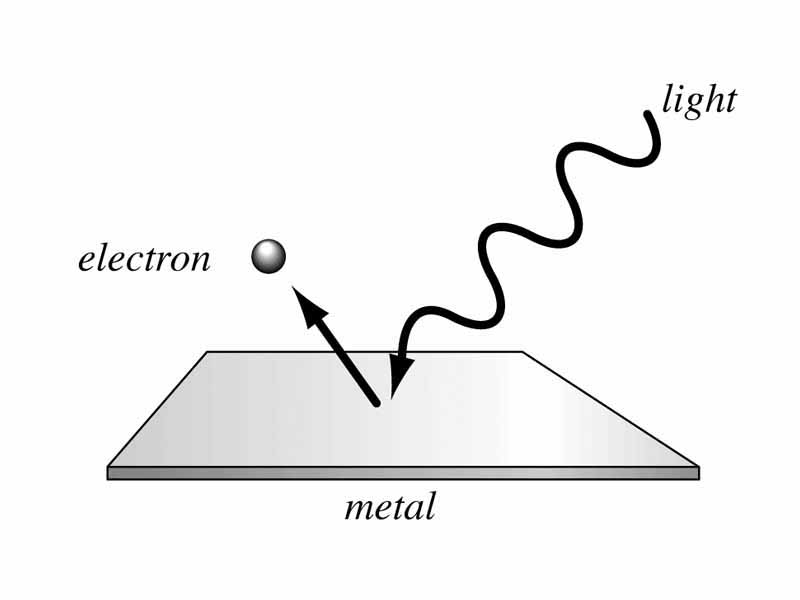# 1. Basics of Quantum Theory

At the most fundamental level, solar cells are nothing more than a large collection of very small, interacting particles. Therefore, the study of solar cells must begin with understanding the basic behavior of small particles. Quantum mechanics is the area of physics that describes interactions among small particles; it is therefore very important to solar cell technology, because it is needed in order to understand how the various particles within the solar cell and solar radiation interact with one another. The field of quantum mechanics is an expansive, fascinating, and ever-growing application of physics. We will deal only with the most basic and relevant principles here.

Quantum theory is the study of very small objects, such as electrons and photons. The study of these small objects differs from classical physics quite drastically. Just as the seemingly smooth images on a computer screen become rough aggregations of single-color pixels if you look close enough, the apparently smooth and continuous world of classical physics transforms into a world of small, discrete, quantized building blocks at small enough scales. A beam of light behaves like a barrage of tiny single-frequency particles (photons), electrons move up through a staircase of discrete energy levels, and thermal vibrations in materials move like free-flying packets of pure momentum (phonons).What is Quantum Theory?

## Quintessential Quantum: the Photoelectric Effect

Beginning with the work of physicist Alexandre Edmond Becquerel in 1839, scientists began studying the effects of shining different lights upon materials. Over the next century, many famous physicists, including Heinrich Hertz, J. J. Thompson, and Phillip Lenard studied what we now know as the photoelectric effect. The photoelectric effect is characterized by the ejection of electrons from a metal surface when light is shined on the surface (see Figure 1 below). The electrons come from within the metal; they are ejected from the material due to the large amount of energy they gain from the absorption of light.Figure $$\PageIndex{1}$$: : Shining a light on metal causes the ejection of an electron. Source: Wetzel, John. “Photoelectric Effect Illustration”. Wikipremed.com. 24 Feb. 2012.

Before the early 20th century, scientists believed light behaved strictly as a wave. However, the photoelectric effect called this model into question. Lenard observed that when he shined a red light on a negatively-charged plate, no electrons were ejected; however, when he shined a blue light on the plate, electrons were ejected (Figure 2). The brightness of the light had no effect on the number of electrons ejected! Since the energy of a wave is related to its amplitude (in this case, brightness) and not its frequency (in this case, color), then one would expect a brighter light to eject more electrons, and light color to be irrelevant. The solution to this paradox was put forth by Albert Einstein, who successfully explained the photoelectric effect by instead thinking of light as made up of particles, tiny discrete packets of energy called quanta, rather than a continuous wave.Figure $$\PageIndex{2}$$: : Shining blue light on the negatively-charged plate causes the ejection of electrons, but the red light doesn't. Image property of SolarWiki.

## Photons

These light particles, called photons, are discrete quanta of light energy, whose energy is associated with their frequency (color) instead of their amplitude (the number present). This explains why the blue light (higher frequency) ejects electrons, while the red light (lower frequency) does not. This also explains why the brightness of the light has no effect. The energy of a photon is given by the following equation, where E is the energy of the photon, f is the light’s frequency and h is Planck’s constant:In SI units, h = 6.626068 x 10-34 m2 kg s-1.

Photons, unlike particles such as electrons, are massless. This is actually the reason that they travel at the fastest speed; to have momentum, massless particles must have infinite velocity, while massive particles can never travel as fast as light!

The quantization of light into photons also illuminates another important point: the quantization of atomic energy levels. That is, the amount of energy required to excite an electron enough to exit the material is a discrete value. One photon of sufficient energy (or frequency) must hit an electron for it to be ejected; multiple photons of lesser energy cannot combine to eject an electron.

The photoelectric effect (one photon in, one electron out) is the central scientific paradigm behind solar energy technology, although advances with such research areas as nanoparticles (see Team Publications) has led to interesting results of multiple electrons per photon.

## The Uncertainty Principle

In addition to light, many other particles like electrons behave both like waves and particles; in other words, the particles have wave-particle duality. One very strange detail of quantum theory, the Heisenberg uncertainty principle, states that the momentum (or velocity) and position of a particle cannot be observed accurately at once. This puzzling dilemma arises because the simple act of observing either the momentum or position a quantum particle will give some amount of energy to the particle, which interferes with the accuracy of the measurement of the other quantity!The above equation means that the product of the uncertainties in x (position) and p (momentum) of a single, non-relativistic particle is greater than or equal to h-bar over two where h-bar is Planck's constant, 1.05 x 10-34 Joule seconds. This means that there is a fundamental limit to our knowledge of a particle's position and velocity - we can never know both of them for sure. This is the root of particles having a probabilistic nature on the quantum scale.